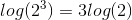# High School Math : Simplifying Logarithms

## Example Questions

### Example Question #1 : Simplifying Logarithms

Simplify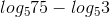.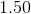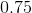Explanation:

Using properties of logs we get: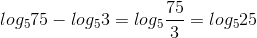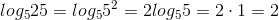### Example Question #2 : Simplifying Logarithms

Simplify the following expression: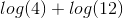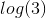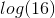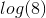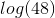Explanation:

Recall the log rule: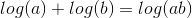In this particular case,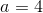and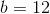. Thus, our answer is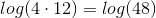.

### Example Question #3 : Simplifying Logarithms

Use the properties of logarithms to solve the following equation: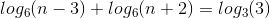No real solutions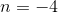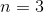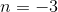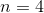Explanation:

Since the bases of the logs are the same and the logarithms are added, the arguments can be multiplied together. We then simplify the right side of the equation: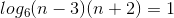The logarithm can be converted to exponential form: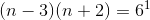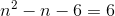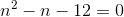Factor the equation: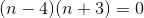Although there are two solutions to the equation, logarithms cannot be negative. Therefore, the only real solution is.

### Example Question #4 : Simplifying Logarithms

Which of the following represents a simplified form of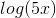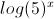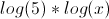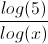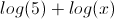Explanation:

The rule for the addition of logarithms is as follows: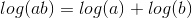As an application of this,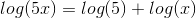### Example Question #1 : Multiplying And Dividing Logarithms

Simplify the expression using logarithmic identities.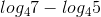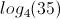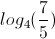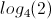The expression cannot be simplified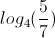Explanation:

The logarithm of a fraction is equal to the logarithm of the numerator minus the logarithm of the denominator.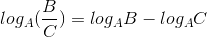If we encounter two logarithms with the same base, we can likely combine them. In this case, we can use the reverse of the above identity.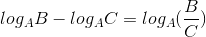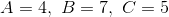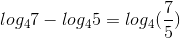### Example Question #6 : Simplifying Logarithms

Evaluate by hand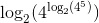Cannot be found by handExplanation:

Using the logarithm rules, exponents within logarithms can be removed and simply multiplied by the remaining logarithm.  This expression can be simplified as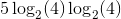### Example Question #7 : Simplifying Logarithms

Solve for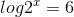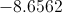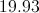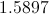Explanation:Use the power reducing theorem: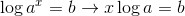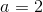and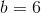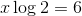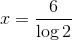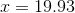### Example Question #8 : Simplifying Logarithms

Which of the following expressions is equivalent to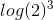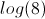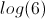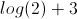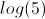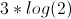Explanation:

According to the rule for exponents of logarithms,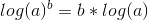. As a direct application of this,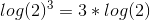.

### Example Question #9 : Simplifying Logarithms

Simplify the expression below.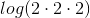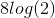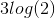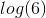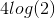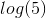Explanation:

Based on the definition of exponents,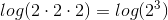.

Then, we use the following rule of logarithms: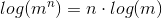Thus,